﻿ Efficiency Analysis of Rice Husk as Adsorbent for Removal of Phenol from Wastewater

# Journal of Environmental & Analytical ToxicologyOpen Access

Like us on:

All submissions of the EM system will be redirected to Online Manuscript Submission System. Authors are requested to submit articles directly to Online Manuscript Submission System of respective journal.
• Research Article
• J Environ Anal Toxicol 2019, Vol 9(3): 605

# Efficiency Analysis of Rice Husk as Adsorbent for Removal of Phenol from Wastewater

Ashanendu Mandal, Paramartha Mukhopadhyay and Sudip Kumar Das*
Department of Chemical Engineering, University of Calcutta, Kolkata, West Bengal, India
*Corresponding Author: Sudip Kumar Das, Department of Chemical Engineering, University of Calcutta, Kolkata, West Bengal, India, Tel: +919830638908, Email: [email protected]

Received Date: Dec 20, 2018 / Accepted Date: May 23, 2019 / Published Date: May 31, 2019

### Abstract

Phenol being a priority pollutant, the purpose of the research is to find out the efficiency of the rice husk as adsorbent for removal of phenol from the wastewater. The characterization of rice husk is studied using scanning electron microscopy, X-ray diffraction, Fourier-transform infrared spectroscopy and Brunauer-Emmett-Teller analyzers. The percentage removal of phenol is obtained in batch experiments with variation of pH (3-9), contact period (60-300 min), adsorbent dosage (2-10 gm/L), phenol concentration (50-250 mg/L) and temperature (25-35°C). The results indicate that the phenol removal reaches up to 95%. The optimized values of adsorption process (pH=7, contact period=240 min, adsorbent dosage=6 gm/L, initial phenol level=50 mg/L and temperature=35°C) are used for testing of various kinetic, thermodynamic and isotherm models. The kinetic study indicates that the pseudo-second order kinetic model (r2=0.99873) is better than the first order kinetic model (r2=0.97984) and hence it supports chemisorptions. The Elovich and the Boyd models also favour chemisorptions. The Dubinin-Radushkevich model supports physisorption, however it is not effective as correlation coefficient (r2=0.73643) is low. The Reichenberg model suggests for film diffusion, while the Fick's model suggests for both and intraparticle diffusion taking 120 min each. The Smith and Furusawa model indicate faster movement of phenol from the bulk to the solid phase. The isotherm study indicates that Freundlich model favors most than Temkin and Langmuir models and hence it supports chemisorptions. The study of thermodynamic supports for spontaneous, endothermic and random process and also supports chemisorption. The novelty of this research is the elaborated analysis of numerous models, which perhaps has not been covered in any of such research work published before. The authors conclude that the rice husk is a low-cost and suitable adsorbent for the removal of phenol from the wastewater.

Keywords: Rice husk; Phenol; Adsorption; Wastewater; Isotherms; Scale-up design

#### Introduction

Phenols are considered as environmental pollutants because of their toxicity . The phenols can cause anorexia, vomiting, fainting, headache and can harm liver and kidney. The United States Environmental Protection Agency and the Indian Ministry of Environment, Forest and climate change have permitted the phenol content in the surface discharge to the maximum extent of 1 mg/L. The World Health Organization has limited phenol content in potable water at 0.001 mg/L.

The phenol and composites of phenol generally get enter in environment while when the effluents from various industries like refinery, plastic, coal tar, disinfectant, pharmaceuticals, steel and rubber plants are released. Phenols are also discharged while the wastewaters from domestic and agricultural run-off are released. The quantum of phenols in the wastewaters may go to as high as 6800 mg/L.

The phenol removal methodologies are usually classified in three groups e.g., chemical, physical and biological . The chemical and biological methods are not preferred as they are expensive and may release toxic elements. The physical methods are now-a-days quite common . Adsorptions are considered to be the most popular physical method and therefore are being studied by many researchers [4-17]. The surface area and pore sizes are assumed to be important factors while selecting the best adsorbent .

The rice husk is chosen as adsorbent in this study since it is highly porous and is having large surface area, hence it can enhance the adsorption efficiency . Further the novelty of the material is that the industry may prefer to use this adsorbent since it is abundantly available all over the world and at almost free of cost. The rice husk used in this research is generally found in the rice mills and is otherwise dumped in rural areas for the uses as fuel or as fodder for domestic cattle. Since the generation of rice husk in the rice mills are plenty and they are collectable at no cost, it’s regeneration as such is not essential . Although some studies are available in the literature for the use of rice husk, however this research work has emphasis on the range of phenol level in wastewater, mostly familiar in many chemical plants.

#### Materials and Methods

In this research work, the adsorbent rice husk is arranged from rice mill near Kolkata, India and washed thoroughly with water. Then it is sun-dried for seven days, crushed to powder in a grinder and sieved through -44 +52 mesh. Since no further treatment is required approximate 1.0 kg of this sieved rice husk with the sizes from 300 to 350 μm is kept in desiccators for the research work. The equipments installed in the Chemical Engineering Department, Calcutta University, viz. Scanning electron microscope (Model S415A, Hitachi), X-ray diffractor (Model D8 Advance, Bruker), Fourier-transform infrared spectroscope (Model NicoletTM 5N, ThermoFisher, USA), Brunauer-Emmett-Teller Analyzer (Model Quantachrome, NOVA 1000e, USA) are used for the surface characterization of the rice husk.

The adsorbate phenol and other reagents (Merck, India) used in this research are of AR grade and arranged from local shop in Kolkata. Initially, 1000 ml of aqueous phenol (1000 ppm) is prepared by mixing 1.0 gm phenol in deionised water. The phenol concentrations as desired are varied by further dilution with distilled water. The residual concentrations of phenol after adsorption are assessed after addition of 4-Amino antipyrene by a spectrophotometer (DR 5000 Hach Co., Germany) installed in the Chemical Engineering Department, University of Calcutta. The pH is measured using pH meter (Multi 304i, Hach Co., Germany) installed in Chemical Engineering Department, University of Calcutta.

25 ml of aqueous phenol solution (1000 ppm) is kept in conical flasks (250 ml). This is diluted by addition of more deionised water to prepare the required phenol level (50-250 mg/L). The pH is varied from 2 to 9 by adding HCl and NaOH. The dose of rice husk is varied from 2 gm/L to 10 gm/L and then the solution is placed in electrically thermostatic shaker for the desired time duration from 60 to 300 min. The temperatures are maintained at 25°C, 30°C and 35°C. After the elapse of specified time duration, the precipitate is filtered out and the resultant solution is measured in the spectrophotometer for the residual quantum of phenol . The experiments are repeated thrice, and the mean is considered for more accuracy.

The removal of phenol (%) is computed from Equation (1).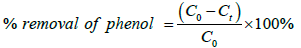(1)

Here C0 and Ct are phenol concentrations (mg/L) in the beginning and after specified time t.

The quantity of phenol adsorbed is computed from Equation (2).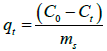(2)

Here qt is phenol adsorbed per unit mass of adsorbent (mg/ gm) at time t and ms is the amount of adsorbent taken (gm/L). The experiments are performed with the change of parameters, e.g., initial pH (2-9), adsorbent dosage (2-10 gm/L), contact duration (60-300 min), temperature (25-35°C) and phenol ppm (50-250 mg/L).

#### Results and Discussion

Characterization of rice husk

The SEM results for the adsorbent is given in Figure 1. It shows that the adsorbent is spongy with the numerous pores. The result of XRD indicating the existence of crystalline silica is shown in Figure 2. The result is displayed in terms of Bragg angle 2θ (10°-80°). The data is compared with the peak values available in Joint Committee of Powder Diffraction Standards  and suggests existence of crystalline silica at 220. The crystalline nature is established due to the presence of sharp peak of crystal phase [9-11]. The high crystallinity in the XRD occurs due to the presence of large quantity of silica in rice husk. The result of FTIR shown in Figure 3 suggests the vibrations of N-H and O-H bonds for the broadband at 3774 cm-1 and 3363 cm-1. The bands 2929-2128 cm-1 are allocated for C-H stretching mode, which signifies the aliphatic nature of the adsorbent. The adsorbent bands of 1658 to 1426 cm-1 indicate the existence of aromatic C=C bonds. The peak of 1080 cm-1 specifies very strong existence of C-O group on surface of bio adsorbent, whereas the peak of 466 cm-1 indicates strong existence of silica (SiO2). The result of BET analyzer indicates that the rice husk has predominately mesopores with the pore diameter between 2 nm and 50 nm with surface area of approximately 320 m2/gm. The elemental analysis and the physical properties of adsorbent are placed at Table 1 . The point of zero charge (pHpzc) is measured using salt adding method  and is estimated at 4.61.

Composition
Constituent % by weight
Carbon 41.44
Hydrogen 4.94
Oxygen 37.32
Nitrogen 0.57
Silicon 14.66
Potassium 0.59
Sodium 0.035
Sulphur 0.3
Phosphorus 0.07
Calcium 0.06
Iron 0.006
Magnesium 0.003
Zinc 0.006
Physical properties
Bulk density, gm/cm3 0.79
BET surface area, m2/gm 320

Table 1: Chemical composition and physical properties of rice husk.

Variation of pH

The pH being an major factor during adsorption process , the study is therefore made with the variation of pH (3-9) but keeping the other process variables constant i.e., at phenol level 50 mg/L, adsorbent dose 6 gm/L, contact duration 240 min and constant temperature 35°C. The experiment is repeated with the variation of phenol level ranging between 50 and 250 mg/L. The graph of phenol removal against pH at Figure 4 shows that phenol removal rises for increase of pH (3-5). However, if the pH is increased further (5-9), the removal decreases substantially. This is because of the amphoteric properties of the adsorbent . At low pH, the removal is less because of the presence of H+ ions preventing phenol ionization. At high pH, the removal increases because of the presence of OH- ions preventing phenolate ions . At further higher pH, the removal again decreases because of the competition between OH- ions and phenolate ions. It also supports the common perception that adsorption favours for pH>pHpzc.

Variation of contact time

The study is performed with the change of contact duration (60-300 min) keeping the other variables constant i.e., at pH of 5, at adsorbent dose of 6 gm/L, at phenol level of 50 mg/L and at temperature of 35°C. This is repeated with the variation of phenol level ranging between 50 and 250 mg/L. The graph of phenol removal against adsorption duration in Figure 5 indicates that the removal is initially more but becomes equilibrium at 240 min. The adsorption is faster initially due to the increased quantity of the unfilled locations of the adsorbent .

The study is performed with the change of the adsorbent dose from 2 to 10 gm/L keeping the other variables constant i.e., at pH of 5, at phenol level of 50 mg/L, at contact duration at 240 min and at temperature of 35°C. This is repeated varying phenol level (50-250 mg/L). The graph of removal of phenol against adsorbent dosage in Figure 6 represents that the equilibrium reaches at 6 gm/L. The removal increases fast in the beginning because of extra adsorption points and thereafter remains stable because of saturation.

Variation of temperature

The study is performed with the change of temperature from 25 to 35°C keeping the other variables constant i.e., at pH of 5, at phenol level 50 mg/L, time duration 240 min and at adsorbent dosage of 6 gm/L. This is repeated with the variation of phenol level (50-250 mg/L). The graph phenol removal against temperature in Figure 7 represents that percentage increases with rise in the temperature (25-35°C), representing higher chemical interaction in phenol with the surface functionality of rice husk. During chemical interaction new type of chemical bonds are generated at the surface of rice husk. The heterogeneous nature of the rice husk facilitates the chemical interaction between the adsorbent and phenol molecules through the exchange of electrons and the valency forces plays major role in such interactions . Since phenol adsorption is endothermic, degree of adsorption rises with increase in temperature.

Variation of phenol level

The studies are performed with the change of phenol level from 50 to 250 mg/L. However, the phenol removal is examined in each study at pH of 5, duration of 240 min, at adsorbent dosage 6 gm/L, at temperature 35°C. This is shown in Figure 8, which exhibits that the phenol removal decreases with increasing phenol level. The removal is less at higher phenol level because of the inadequacy of the total adsorption sites after gathering of phenol .

Kinetic study

In our experimental data analysis, we have tested six kinetic models.

Pseudo-first order model: The model  is given by the Equation (3).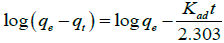(3)

Here qe and qt denote the quantum of adsorbed phenol (mg/gm) at equilibrium and at time t, and Kad is kinetic rate constant (min-1). This model supports for physisorption and homogeneous adsorbent .

In the graph of log(qe-qt) against t, correlation coefficient (r2) and Kad are found to be 0.97984 and 0.00967 (min-1).

Pseudo-second order model: This model is stated in the Equation (4).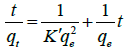(4)

Here qe and qt denote the quantum of adsorbed phenol (mg/gm) at equilibrium and at time t, and K' is kinetic rate constant (gm/mg min). This model supports for chemisorption and heterogeneous adsorbent .

From the graph of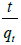versus t, the correlation coefficient (r2) and K' are found to be 0.99991 and 0.006565 gm/mg min respectively.

Table 2 shows that there is better agreement in the calculated and the experimental qe values in favour of the pseudo-second order kinetics. This suggests for chemisorptions and heterogeneous.

Co (mg/L) qe, exp (mg/gm) Lagergen first order Pseudo second order
Kad (1/min) qe, cal (mg/gm) r2 K' (gm/mg min) qe, cal (mg/gm) r2
50 7.89 0.0096726 2.274 0.97984 0.0065653 8.38 0.99873

Table 2: Kinetic parameters of Pseudo first-order and pseudo second-order models.

Elovich model: The model is given in the Equation (5).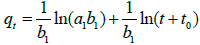(5)

Here qt denotes the quantum of adsorbed phenol (mg/gm) at time t and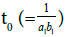are Elovich constants. The graph against shows the high correlation coefficient of 0.96619 and therefore it indicates that process of the adsorption is chemical .

Boyd model: The model is given in the Equation (6) .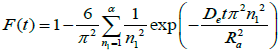(6)

For spherical adsorbent particles, Vermeulen simplifies the Equation (6) by Equation (7).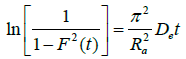(7)

Here F(t) denotes the proportion of phenol adsorbed in adsorbent at time t to that at the equilibrium time, Ra denotes radius of adsorbent particles (m), and De denotes the effective diffusion coefficient (m2/sec) of the adsorbate. The graph of with t gives the correlation coefficient of 0.97620. From the slope the diffusion coefficient is computed at 1.6444 × 10-12 m2/sec. Since the value of De is in range of 10-9-10-17 m2/sec, it supports for chemisorptions system .

Reichenberg model: The model  is given in the Equation (8).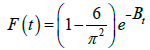(8)

The Equation (8) is further simplified as in the Equation (9).

Bt=-0.4977ln[1-F(t)]                (9)

Here F(t) denotes the proportion of phenol adsorbed in adsorbent at time t to that at the equilibrium time and Bt is the time dependent constant. The plot of Bt and t indicates that the correlation coefficient (r2) is high (0.97984) and hence supports film diffusion.

Fick model: The model is given in Equation (10).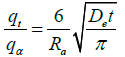(10)

Here qα (assumed as qe) is quantum of phenol (mg) per unit of adsorbent (gm) at equilibrium, Ra denotes radius of adsorbent particles (m), and De denotes the effective diffusion coefficient (m2/sec) of the adsorbate. The graph of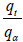and √t implies that the first linear portion indicates film diffusion which remains for first 120 min, the second linear portion indicates intraparticle diffusion which remains for next 120 min and final line indicates stability which occurs at 240 min. Therefore, the film and intraparticle diffusion in batch experiment has ratio 1:1.

The results of these statistical models are shown in Table 3 which confirms their fine conformity because of higher correlation coefficients.

Kinetic model Model equation Correlation coefficient Result
Elovich model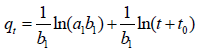0.96619 Adsorption is chemical
Boyd model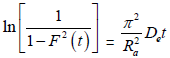0.9762 Supports for chemisorption
Reichenberg model Bt=-0.4977ln[1-F(t)] 0.97984 Adsorption is of film diffusion
Fick model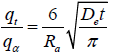1.0 The film diffusion and the intraparticle diffusion take about 120 min and 240 min

Table 3: Various kinetic model parameters.

Isothermal study

Langmuir isotherm: The model is stated in Equation (11).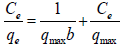(11)

Here, Ce denotes phenol level at the equilibrium (mg/L), qe denotes quantum of phenol (mg) per unit of adsorbent (gm) at equilibrium, qmax (mg/gm) and b (L/mg) are Langmuir constants. From the plot of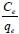against Ce, the qmax and b are computed  and are given in Table 4. The qmax signifies that this quantity of phenol in rice husk (gm) forms monolayer and b signifies for strong bonding with the adsorbent . The Langmuir isotherm also evaluates RL, the constant separation factor (dimensionless), as in Equation (12).

Langmuir isotherm Freundlich isotherm Temkin isotherm
qmax (mg/gm) b (L/mg) r2 Kf n r2 KT B1 r2
50.15 0.0575 0.96921 4.5583 1.7533 0.99865 6.06783 2.97175 0.9816

Table 4: Various isotherm model parameters.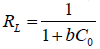(12)

Applying b, RL are calculated as 0.258, 0.148, 0.104, 0.080 and 0.065 at the phenol level of 50, 100, 150, 200 and 250 mg/L respectively. Since the values of RL lie between 0 and 1, Langmuir isotherm is favorable .

Freundlich isotherm: The model is stated by Equation (13).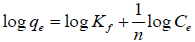(13)

Here, Ce denotes phenol level at the equilibrium (mg/L), qe denotes quantum of phenol (mg) per unit of adsorbent (gm) at equilibrium, Kf and n are the Freundlich constants. From the plot of log qe and log Ce, the Kf and n are computed  and their values are given in Table 4. Here Kf represents for quick removal and n represents heterogeneous since the limit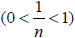is followed .

Temkin isotherm: The model is given by Equation (14).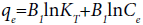(14)

Here, Ce denotes phenol level at the equilibrium (mg/L), qe denotes quantum of phenol (mg) per unit of adsorbent (gm) at equilibrium, and B1 and KT are constants related to equilibrium binding (L/gm) and adsorption heat (J/mol) respectively. The graph qe against ln Ce evaluates the Temkin constants B1 and KT which are given in Table 4. The B1 and KT signify that adsorption process is chemical.

Table 4 indicates that all three isotherm models are applicable due to higher correlation coefficients (r2), however Freundlich isotherm is the best supportive one due to its highest correlation coefficient.

Analytical study

For any adsorption process, it is essential to find out the extent of phenol transport from the bulk stage to rice husk surface and for predicting the physisorption or chemisorption. Several analytical models have been published in the literature, of which Furusawa and Smith model and Dubinin-Radushkevich model are used here.

Furusawa and Smith model: The model estimates the external mass transfer on rate of adsorption and is stated as Equation (15).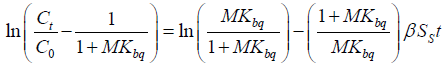(15)

Here C0 and Ct denote the phenol level (mg/L) initially and at time t, M denotes mass of adsorbent (gm/L), β denotes coefficient of mass transfer (cm/sec), SS denotes external surface area per unit volume (m-1), and Kbq denotes constant (L/gm). The Kbq is calculated by multiplying b with qmax computed from Langmuir Isotherm. The graph of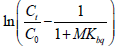against t shows the correlation coefficient of 0.97940 and the coefficient of mass transfer (β) of 6.085 × 10-11, which suggests that the phenol shifts quicker from bulk to solid .

Dubinin-Radushkevich model: The model is stated by Equation (16).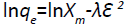(16)

Here qe denotes phenol adsorbed on surface of adsorbent (mg/ gm), Xm denotes maximum capacity of adsorption (mg/gm), λ denotes adsorption energy constant (square moles/square KJ) and ε denotes Polanyi potential (square KJ/square mole) computed from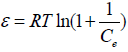.

In graph ln qe against Ɛ2 for correlation coefficient of 0.73643, λ and Xm are calculated as 1.81936 (square moles per square KJ) and 26.02688 (mill moles/gm). Further adsorption energy (E) is computed as 0.5242 KJ/mole from Equation (17).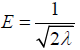(17)

Since E>8 KJ/mole, the adsorption supports physisorption. But this is not applicable due to low value of correlation coefficient.

Thermodynamic study

The thermodynamic study involves Equations (18) and (19).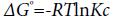(18)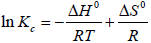(19)

Here, ΔG0, ΔH0, ΔS0 denote Gibbs free energy (KJ/mole), enthalpy (KJ/mole) and entropy (KJ/mole K), R denotes ideal gas constant (0.008314 KJ/mole K) and Kc denotes equilibrium constant. The values of ΔG0, ΔH0, ΔS0 at 25°C, 30°C and 35°C are calculated and placed at Table 5. Here ΔGo being negative supports for spontaneous adsorption, and ΔHo, ΔSo being positive suggest random and endothermic adsorption. Further greater values of ΔGo with rise of temperature and positive value of ΔHo are also supportive of experimental result showing increased removal with temperature.

T (0K) ΔG0 (kJ/mole) ΔH0 (kJ/mole) ΔS0 (kJ/mole Kelvin)
298 -0.01739 84.134 0.282
303 -1.02142
308 -2.84585

Table 5: Values of ΔGo, ΔHo and ΔSo.

#### Safe Disposal of Used Adsorbent

The adsorbent rice husk is arranged from rice mill at Kolkata, India. This is not hazardous and therefore no treatment is required before use in the research. The availability of rice husk does not have any shortage anytime since there are plenty of rice mills running all over the world, especially in the Asian countries. The rice husk is also very cheap and therefore its regeneration as such does not arise. The toxicity of adsorbed phenol in the rice husk can be easily destroyed by incineration and therefore can be used safely after incineration for road repairing, land refilling, etc.

#### Scale Up Design

The design for the batch adsorption generally involves the adsorption isotherm . The scale-up design using the concept of mass balance as in Equation (20).

The mass balance equation is given by Equation (20).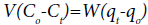(20)

Here W denotes mass of rice husk (gm), V denotes volume of aqueous phenol solution (L), Co and Ct denote phenol concentration initially and at time t (mg/L), qo and qt denote phenol adsorbed on surface of rice husk initially and at time t (mg/gm).

Initially when t=0, q0=0 and at equilibrium Ct=Ce and qt=qe. Therefore, the Equation (20) is modified as Equation (21).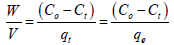(21)

Using the best supportive isotherm model (Freundlich model), the Equation (19) is changed as Equation (22).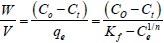(22)

Here n and Kf are the Freundlich constants.

Using Equation (22), quantum of rice husk (gm) for the phenol solution (2-10 L) is calculated and is shown in the Table 6.

2 28.38 21.29 14.19 7.10
4 56.76 42.57 28.38 14.19
6 85.14 63.86 42.57 21.29
8 113.53 85.14 56.76 28.38
10 141.91 106.43 70.95 35.48

Table 6: Weight of the adsorbent (gm) for different volume of phenol solution (L).

#### Conclusion

The removal of phenol using rice husk as adsorbent is studied. The adsorbent is characterized by SEM, FTIR, XRD and BET machines. The impacts of pH, contact duration, adsorbent dose, temperature and phenol level for adsorption are examined. The equilibrium reaches at pH 5, duration of 240 min, dose 6 gm. The phenol removal decreases with increase of phenol concentration (50-250 mg/L) and increases with increase of temperature (25-35°C). The pseudo-second order is better and hence supports chemisorptions. The Elovich model and the Boyd model also support chemisorptions. While Reichenberg model signifies film diffusion, Furusawa and Smith model suggests for fast movement of phenol from solution to solid. The Fick model describes that both film diffusion and intraparticle diffusion take 120 min each. The adsorption energy calculation indicates that adsorption is described by physisorption, however it is not effective due to low correlation coefficient. The Freundlich isotherm favours most than Langmuir and Temkin isotherms. The study of thermodynamic suggests that the process is spontaneous, random and endothermic. The research concludes that rice husk is suitable adsorbent for removal of phenol from the wastewater. The range of initial phenol concentration considered in the research supports its application in petroleum refineries and many other allied industries.

#### Conflict of Interest

The authors have no conflicts of interest to disclose.

#### References

Citation: Mandal A, Mukhopadhyay P, Das SK (2019) Efficiency Analysis of Rice Husk as Adsorbent for Removal of Phenol from Wastewater. J Environ Anal Toxicol 9: 605.

Copyright: © 2019 Mandal A, et al. This is an open-access article distributed under the terms of the Creative Commons Attribution License, which permits unrestricted use, distribution, and reproduction in any medium, provided the original author and source are credited.

Select your language of interest to view the total content in your interested language

##### Recommended Journals
Viewmore
###### Article Usage
• Total views: 268
• [From(publication date): 0-0 - Nov 18, 2019]
• Breakdown by view type
• HTML page views: 235Can't read the image? click here to refresh